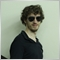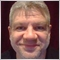# Which is the best/proper basic code structure of an indicator's OnCalculate function?2321

Hi!

Most of the time, custom indicators will require some basic structure with code that are so common that can be used for ctrl+c/ctrl+v purposes. Up until now, the basic structure I've been using for the OnCalculate is as follows:

```int OnCalculate(const int rates_total,
const int prev_calculated,
const datetime &time[],
const double &open[],
const double &high[],
const double &low[],
const double &close[],
const long &tick_volume[],
const long &volume[],
const int &spread[])
{
int startPos;
bool isNewCandleRT = false;

const bool isCalculatingForNow = prev_calculated == rates_total;

if (prev_calculated == 0)
{
if (Bars(_Symbol,_Period) < 2)
return 0;

if (BarsCalculated(glHandleFastMA) < 0)
return 0;

startPos = 1;

PlotIndexSetInteger(0,PLOT_DRAW_BEGIN,startPos);
PlotIndexSetInteger(1,PLOT_DRAW_BEGIN,startPos);
PlotIndexSetInteger(2,PLOT_DRAW_BEGIN,startPos);

//Etc.
}
else if (isCalculatingForNow)
{
//Print("Is now");
startPos = MathMax(prev_calculated - 1,1);
}
else
{
//Print("New candle produced");
startPos = prev_calculated;
isNewCandleRT = true;

//Etc.
}

//
double dataTemp[];

for (int aaa = startPos; aaa < rates_total && !IsStopped(); ++aaa)
{
if (CopyBuffer(glHandleFastMA,0,rates_total - aaa - 1,1,dataTemp) < 0)
{
Print("Error copying fast moving average data (error: ",GetLastError(),")");

continue;
}

//Etc.
}

//--- return value of prev_calculated for next call
return rates_total;
}```

And this has worked for me so far. But recently I was researching over an occurance of error 4806 and found this topic which seems to suggest that when calling for CopyBuffer inside OnCalculate, it would be better not to iterate for each candle at the indicators' start as the code above. Rather, the 'proper' way would be to call for a single CopyBuffer for the entire data and then proceed with single candle CopyBuffer for current real time data.

That observation (if I understood it correctly) led me to two questions:
1) Is this approach correct? Should I change my default code above to something like:

```int OnCalculate(const int rates_total,
const int prev_calculated,
const datetime &time[],
const double &open[],
const double &high[],
const double &low[],
const double &close[],
const long &tick_volume[],
const long &volume[],
const int &spread[])
{
int startPos;
bool isNewCandleRT = false;

const bool isCalculatingForNow = prev_calculated == rates_total;

if (prev_calculated == 0)
{
if (Bars(_Symbol,_Period) < 2)
return 0;

if (BarsCalculated(glHandleFastMA) < 0)
return 0;

startPos = 1;

PlotIndexSetInteger(0,PLOT_DRAW_BEGIN,startPos);
PlotIndexSetInteger(1,PLOT_DRAW_BEGIN,startPos);
PlotIndexSetInteger(2,PLOT_DRAW_BEGIN,startPos);

//Etc.
double dataTemp[];

if (CopyBuffer(glHandleFastMA,0,rates_total - startPos - 1,rates_total - 1,dataTemp) < 0)
{
Print("Error copying fast moving average data (error: ",GetLastError(),")");

continue;
}

for (int aaa = startPos; aaa < rates_total && !IsStopped(); ++aaa)
{
//Do something with dataTemp[aaa];
}

return rates_total;
}
else if (isCalculatingForNow)
{
//Print("Is now");
startPos = MathMax(prev_calculated - 1,1);
}
else
{
//Print("New candle produced");
startPos = prev_calculated;
isNewCandleRT = true;

//Etc.
}

//
for (int aaa = startPos; aaa < rates_total && !IsStopped(); ++aaa)
{
if (CopyBuffer(glHandleFastMA,0,rates_total - aaa - 1,1,dataTemp) < 0)
{
Print("Error copying fast moving average data (error: ",GetLastError(),")");

continue;
}

//Etc.
}

//--- return value of prev_calculated for next call
return rates_total;
}```

2) Since I'm already revising my basic copy-paste code for all new indicators I make, I might extend the scope of my problem to: which is the best/proper basic code structure of an indicator's OnCalculate function?41889

Martin Bittencourt:

Hi!

Most of the time, custom indicators will require some basic structure with code that are so common that can be used for ctrl+c/ctrl+v purposes. Up until now, the basic structure I've been using for the OnCalculate is as follows:

And this has worked for me so far. But recently I was researching over an occurance of error 4806 and found this topic which seems to suggest that when calling for CopyBuffer inside OnCalculate, it would be better not to iterate for each candle at the indicators' start as the code above. Rather, the 'proper' way would be to call for a single CopyBuffer for the entire data and then proceed with single candle CopyBuffer for current real time data.

That observation (if I understood it correctly) led me to two questions:
1) Is this approach correct? Should I change my default code above to something like:

Yes it is. Your initial approach is inefficient.

2) Since I'm already revising my basic copy-paste code for all new indicators I make, I might extend the scope of my problem to: which is the best/proper basic code structure of an indicator's OnCalculate function?

This question way to general.

For example your default code doesn't suit my needs. So it all depends.2321

Alain Verleyen:

Yes it is. Your initial approach is inefficient.

This question way to general.

For example your default code doesn't suit my needs. So it all depends.

1) Thank you!

2) Well, little tips suffice. For example: the lines

```if (Bars(_Symbol,_Period) < 2)
return 0;```

are now included in all my indicators as a result of loading MT5 with indicators attached while the data wasn't fillied in, resulting in crash. So if someone would make me the question I made, I'ld say to them "include these lines to prevent this common problem".

With that in mind, are there any smart, generic code lines to be included apart from the ones I'm already using? :)